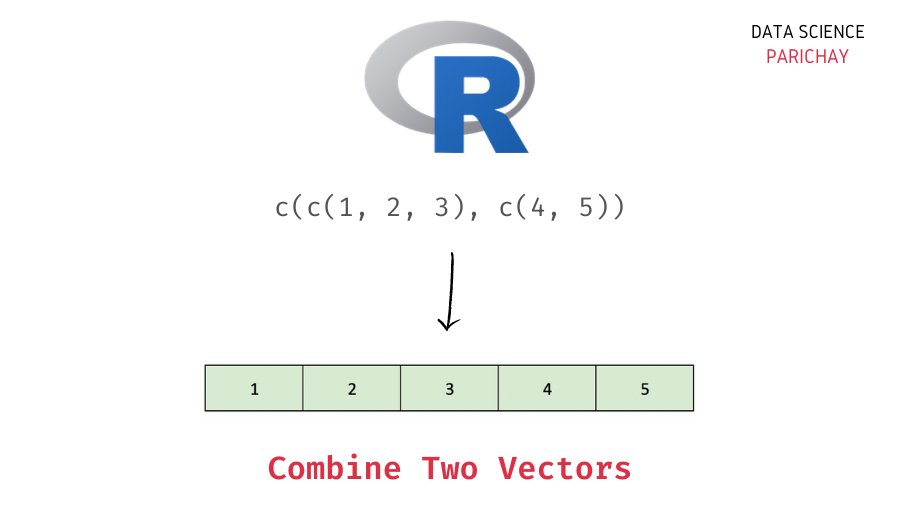# Combine Two Vectors Into a Single Vector in R

In this tutorial, we will look at how to combine two vectors into a single vector in R with the help of some examples.

## How to combine two vectors in R?

You can use the `c()` function in R to combine two or more vectors into a single vector. Pass the vectors you want to combine as comma-separated arguments to the `c()` function. The following is the syntax –

```# combine vectors
c(vec1, vec2, vec3, ...)```

It returns a single combined vector.

## Examples

Let’s look at some examples of combining vectors.

### Combine two numeric vectors in R

Let’s combine two numeric vectors together into a single vector. First, we will use the `c()` function to create the individual vectors and then use the `c()` again to combine the vectors together.

```# combine two numeric vectors
vec <- c(c(1, 2, 3), c(4, 5))
# display the resulting vector
print(vec)```

Output:

` 1 2 3 4 5`

We get a single vector with values combined from both vectors.

Note that the `c()` function on all occasions in the above example is performing the same task – combining values (or vectors) together. Let’s look at this in more detail.

📚 Data Science Programs By Skill Level

Introductory

Intermediate ⭐⭐⭐

🔎 Find Data Science Programs 👨‍💻 111,889 already enrolled

Disclaimer: Data Science Parichay is reader supported. When you purchase a course through a link on this site, we may earn a small commission at no additional cost to you. Earned commissions help support this website and its team of writers.

• We use the `c()` function to create two vectors using `c(1, 2, 3)` and `c(4, 5)`. The function here is taking these individual values and then combining them into a vector. An individual value, for example, `3` can be treated as a vector of length one. Thus, you can think of `c(1, 2, 3)` as combining three vectors of length one into a single vector of length 3.
• The `c()` function is then used to combine the two vectors into a single vector of length five.

### Combine two character vectors in R

Let’s now combine two vectors with character type values using the `c()` function.

```# combine two character vectors
vec <- c(c("a", "b", "c"), c("d", "e"))
# display the resulting vector
print(vec)```

Output:

` "a" "b" "c" "d" "e"`

Here, we combine vectors `c("a", "b", "c")` and `c("d", "e")` into a single character vector of length five.

### Combine two vectors with different value types

Vectors store values of the same type in R. When you combine vectors with values of different datatypes, R performs internal coercion such that the values are consistent with the same data type.

The following is the priority order for data type conversion –
`logical < numeric < complex < characters`

Let’s look at an example. Here, we will combine a numeric vector with a character vector.

```vec <- c(c(1, 2, 3), c("a", "b", "c"))
# display the resulting vector
print(vec)```

Output:

` "1" "2" "3" "a" "b" "c"`

You can see that the resulting vector contains all the values from both the vectors but the values are converted to the character type.

You might also be interested in –

•